• 基于BP神经网络原理，针对数据当中所需的分类需求进行开发的小程序，具备一定的直到作用。
• 网上找到的资源，代码比较基础，内有自带数据，可运行，大家可以下下来进行学习。
• 01-BP神经网络-分类,bp神经网络二分类,matlab源码.zip
• 吴恩达网易云公开课《深度学习》week3--shallow_nn_model二分类
• 今天小编就为大家分享一篇Pytorch实现神经网络分类方式，具有很好的参考价值，希望对大家有所帮助。一起跟随小编过来看看吧
• 本例采用BP神经网络，对鸢尾花数据做分类，实现比较高的分类准确率
• 题目： 企业到金融商业机构贷款，金融商业机构需要对企业进行评估...编写matlab代码，构造一个输入结点为2，中间隐藏层结点为5，输出结点为1的BP神经网络。其训练次数设置为200，训练目标最小误差设置为0.00001。用训练
题目： 企业到金融商业机构贷款，金融商业机构需要对企业进行评估。评估结果为0和1两种形式，0表示企业两年后破产，将拒绝贷款；而1表示企业2年后具备还款能力，可以贷款。表5-9中，已知前20家企业的三项评价指标值和评估结果，试建立神经网络，决策树模型对剩余5家企业进行评估。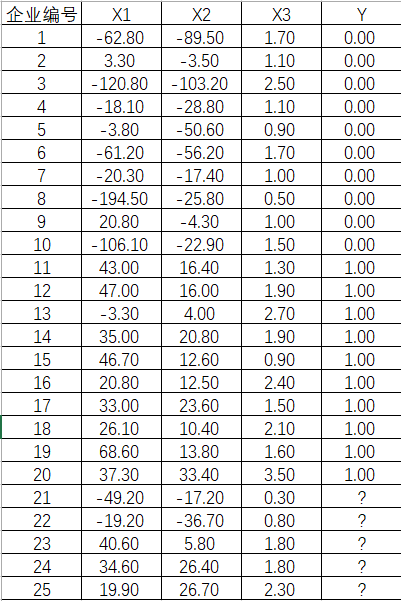(数据下载，无需积分)
解题过程：
建立双层前向神经网络模型：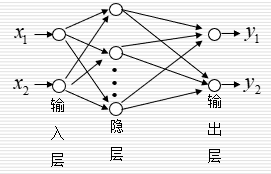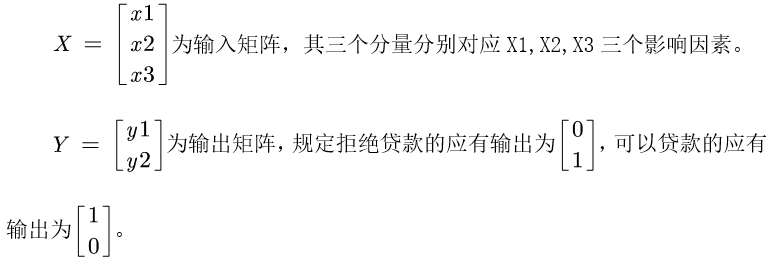编写matlab代码，构造一个输入结点为2，中间隐藏层结点为5，输出结点为1的BP神经网络。其训练次数设置为200，训练目标最小误差设置为0.00001。用训练样本对BP神经网络进行训练。训练效果如下：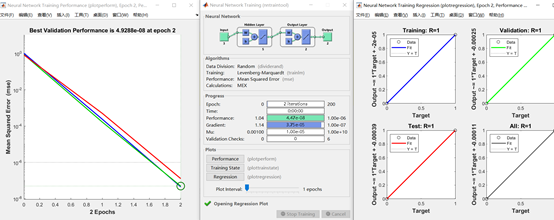检验样本的预测值（红线）和期望值（蓝线）如下所示：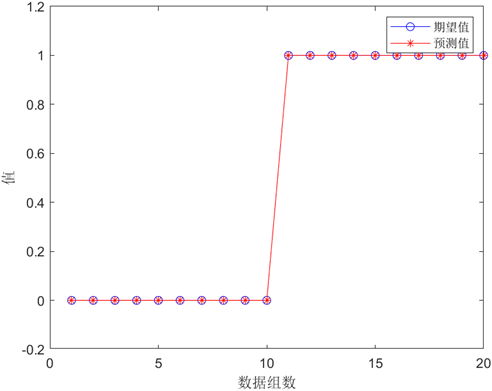将题目中未分类的五组数据作为预测集输入到我们构建的BP神经网络中，得到预测结果如下：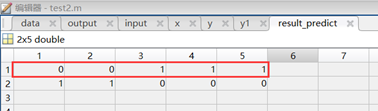因此，利用BP神经网络我们预测出以下结论：编号为21、22的企业银行将拒绝贷款，编号为23,24,25的企业银行可以贷款。
代码如下：
%% 清空环境变量
clear
clc
%% 读取数据
input = data(1:25,2:4)';%载入输入数据
output = data(1:20,5)'; %载入输出数据
x = input(:,1:20);
[xn,xps]=mapminmax(x);%归一化到[-1,1]之间
y = zeros(2,20);
for i=1:20
if output(1,i)==1
y(1,i)=1;
y(2,i)=0;
else
y(1,i)=0;
y(2,i)=1;
end
end
%% 建立两层前向神经网络
net=newff(xn,y,5,{'tansig','purelin'},'trainlm');
net.trainParam.goal=0.000001; %设定训练误差
net.trainParam.epochs=200;  %设定最大训练步数
net=train(net,x,y); %训练网络
y1=sim(net,x); %输出数据
%yy=sim(net,[1.24 1.28 1.40;1.80 1.84 2.04]-1)
%% 数据泛化
input_predict = input(:,21:25);
[input_predictn,input_predictps]=mapminmax(input_predict);%归一化到[-1,1]之间
result_predict = sim(net,input_predictn);
result_predict = round(result_predict);
%% 作图
figure(1)
yy = y(1,:);
y11 = y1(1,:);
plot(yy,'bo-')
hold on
plot(y11,'r*-')
% hold on
% plot(error,'square','MarkerFaceColor','b')
legend('期望值','预测值')
xlabel('数据组数')
ylabel('值')

展开全文数学建模 机器学习 matlab
• 二分类BP算法代码及注释 import numpy as np import matplotlib.pyplot as plt # 中文、负号 plt.rcParams['font.sans-serif'] = ['SimHei'] plt.rcParams['axes.unicode_minus'] = False #数据集 x1 = [0.697,0....
二分类BP算法代码及注释
import numpy as np
import matplotlib.pyplot as plt

# 中文、负号
plt.rcParams['font.sans-serif'] = ['SimHei']
plt.rcParams['axes.unicode_minus'] = False

#数据集
x1 = [0.697,0.774,0.634,0.608,0.556,0.403,0.481,0.437,0.666,0.243,0.245,0.343,0.639,0.657,0.360,0.593,0.719]
x2 = [0.460,0.376,0.264,0.318,0.215,0.237,0.149,0.211,0.091,0.267,0.057,0.099,0.161,0.198,0.370,0.042,0.103]
y = [1,1,1,1,1,1,1,1,0,0,0,0,0,0,0,0,0]

#数据提取
x = np.c_[x1,x2]

# 数据预处理
def preProcess(x,y):
# 特征缩放(标准化)
x -= np.mean(x,0)
x /= np.std(x,0,ddof=1)

# 数据初始化
x = np.c_[np.ones(len(x)),x]
y = np.c_[y]
return x,y
#调用预处理函数
x,y = preProcess(x,y)

# 洗牌
np.random.seed(7)
order = np.random.permutation(len(x))
x = x[order]
y = y[order]

#数据集切分(train/test)
train_x,test_x = np.split(x,[int(0.7*len(x))])
train_y,test_y = np.split(y,[int(0.7*len(x))])

# 正向传播算法
# 激活函数(逻辑函数)
def g(z,deriv=False):
if deriv:
return z*(1-z)
return 1/(1+np.exp(-z))

# 定义模型
def model(x,theta1,theta2,theta3):
z2 = np.dot(x,theta1)
a2 = g(z2)
z3 = np.dot(a2, theta2)
a3 = g(z3)
z4 = np.dot(a3, theta3)
a4 = g(z4)
return a2,a3,a4

# 代价函数
def costFunc(h,y):
j = (-1/len(y))*np.sum(y*np.log(h) + (1-y)*np.log(1-h))
return j

# 向后传播(BP算法)
def BP(a1,a2,a3,a4,theta1,theta2,theta3,alpha,y):
# 求delta值
delta4 = a4 - y
delta3 = np.dot(delta4,theta3.T)*g(a3,True)
delta2 = np.dot(delta3,theta2.T)*g(a2,True)

# 求deltaTheta
deltatheta3 = (1/len(y))*np.dot(a3.T,delta4)
deltatheta2 = (1/len(y))*np.dot(a2.T,delta3)
deltatheta1 = (1/len(y))*np.dot(a1.T,delta2)

# 更新theta
theta1 -= alpha*deltatheta1
theta2 -= alpha*deltatheta2
theta3 -= alpha*deltatheta3
return theta1,theta2,theta3

# 梯度下降函数
m,n = x.shape
k = y.shape

# 初始化theta
theta1 = 2 * np.random.rand(n,hidden_layer_size) - 1
theta2 = 2 * np.random.rand(hidden_layer_size,hidden_layer_size) - 1
theta3 = 2 * np.random.rand(hidden_layer_size,k) - 1

# 初始化代价
j_history = np.zeros(max_iter)

for i in range(max_iter):
# 求预测值
a2, a3, a4 = model(x,theta1,theta2,theta3)
# 记录代价
j_history[i] = costFunc(a4,y)
# 反向传播，更新参数
theta1, theta2, theta3 = BP(x, a2, a3, a4, theta1, theta2, theta3, alpha, y)
return j_history,theta1, theta2, theta3

#调用梯度下降函数

#训练集预测值
train_a2,train_a3,train_h = model(train_x,theta1, theta2, theta3)
#预测结果标签
test_a2,test_a3,test_h = model(test_x,theta1, theta2, theta3)

#定义准确率
def score(h,y):
count = 0
for i in range(len(y)):
if np.where(h[i] >= 0.5,1,0) == y[i]:
count += 1
return count/len(y)

print('训练集准确率:',score(train_h,train_y))
print('测试集准确率:',score(test_h,test_y))

# 画图
plt.title('代价函数图像')
plt.plot(j_history)
plt.show()


效果展示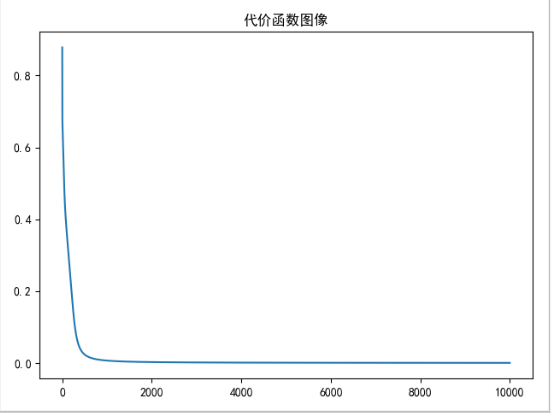展开全文机器学习 有监督学习
• 一、BP神经网络概述 BP神经网络是一种多层前馈神经网络，该网络的主要特点是信号前向传递，误差反向传播。在前向传播的过程中，输入信号从输入层经隐含层处理，直至输出层。每一层的神经元状态只影响下一层神经元...
一、BP神经网络概述
BP神经网络是一种多层前馈神经网络，该网络的主要特点是信号前向传递，误差反向传播。在前向传播的过程中，输入信号从输入层经隐含层处理，直至输出层。每一层的神经元状态只影响下一层神经元状态。如果输出层得不到期望输出，则转入反向传播，根据预测误差调整网络权值和阈值，从而使BP神经网络预测输出不断逼近期望输出。
BP神经网络的拓扑结构如图1-1所示：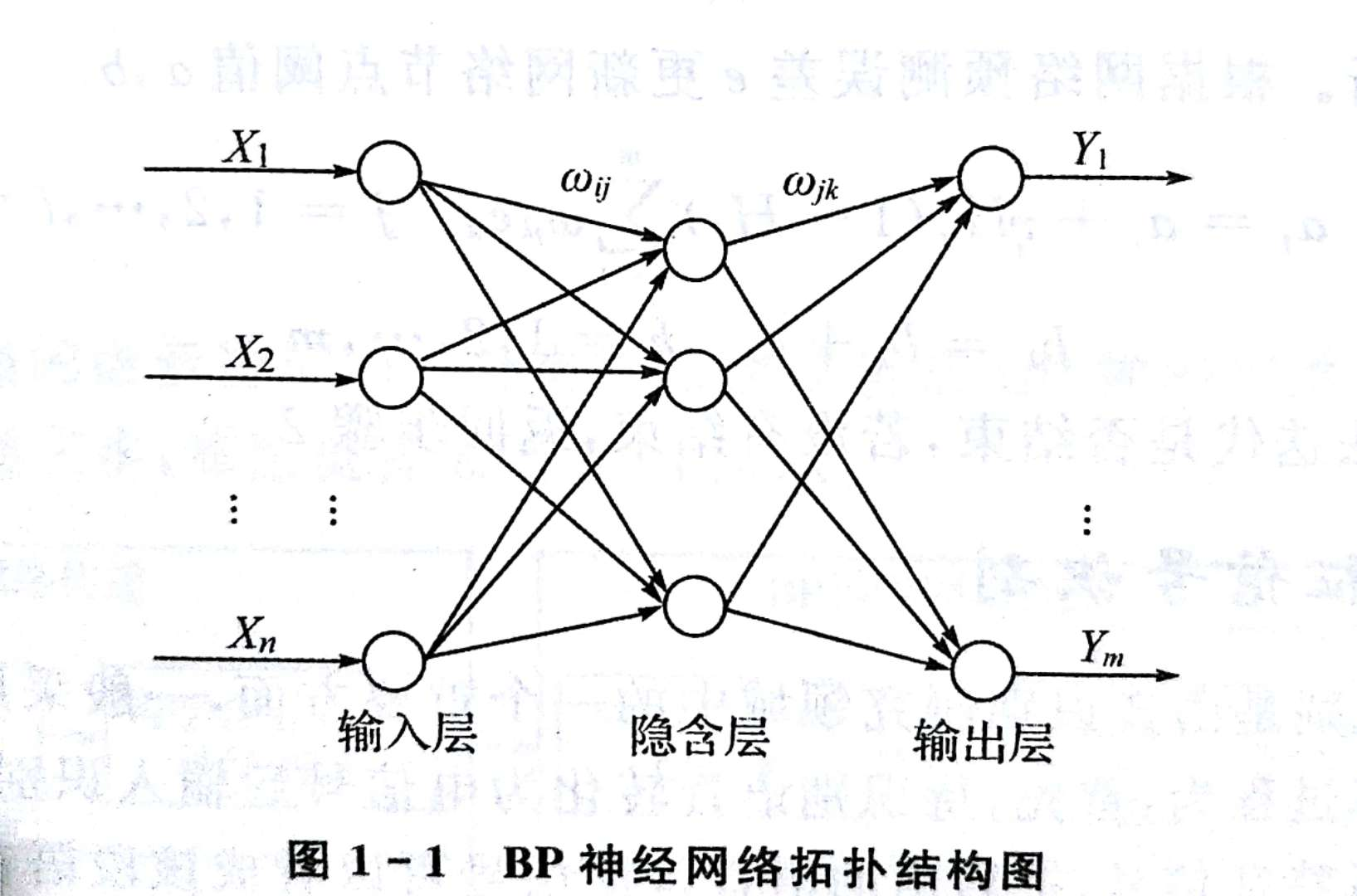其中，X1，X2，...，Xn是BP网络的输入值，Y1，Y2，...，Yn是BP神经网络的预测值，和为BP神经网络的权值。
BP神经网络预测前首先要训练网络，通过训练使网络具有联想记忆和预测能力。BP神经网络的训练过程包括以下几个步骤：
步骤1：网络初始化。根据系统输入输出序列（X，Y）确定网络输入层节点数n、隐含层节点数l，输出层节点数m，初始化输入层、隐含层和输出层神经元之间的连接权值和，初始化隐含层阈值a，输出层阈值b，给定学习速率和神经元激励函数。
步骤2：隐含层输出计算。根据输入变量X，输入层和隐含层连接权值以及隐含层阈值a，计算隐含层输出H。

式中，l为隐含层节点数，f为隐含层激励函数，该函数有多种表达形式。我们使用sigmoid函数

步骤3：输出层计算。根据隐含层输出H，连接权重和阈值b，计算BP神经网络预测输出O。
k = 1,2,...,m
步骤4：误差计算。根据网络预测输出O和期望输出Y，计算网络预测误差e。
k = 1,2,...,m
步骤5：权值更新。根据网络预测误差 e更新网络连接权值，。
i = 1,2,...,l;k = 1,2,...,m
j = 1,2,...,l;k = 1,2,...,m
式中，为学习率。
步骤6：阈值更新。根据网络预测误差e更新节点阈值a，b。
j = 1,2,...,l
k = 1,2,...,m
步骤7：判断算法迭代是否结束，若没有结束，返回步骤2.
二、语音特征信号识别
语音特征信号识别是语音识别研究领域中的一个重要方面，语音识别的运算过程为：首先，待识别语音转化为电信号后输入识别系统，经过预处理后用数学方法提取语音特征信号，提取出的语音特征信号可以堪称该段语音的模式；然后，将该段语音模型同已知参考模式相比较，获得最佳匹配的参考模式为该段语音的识别结果。语音识别流程图如下：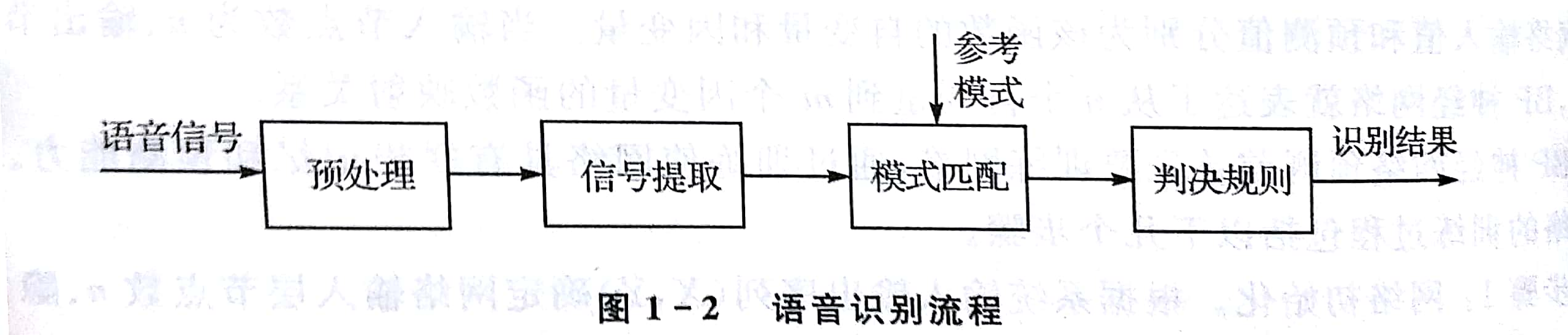本案例选取了民歌、古筝、摇滚和流行四类不同音乐，用BP神经网路实现对这四类音乐的有效分类。每段音乐都用倒谱系数法提取500组24维语音特征信号，提取出的语音特征信号如图所示：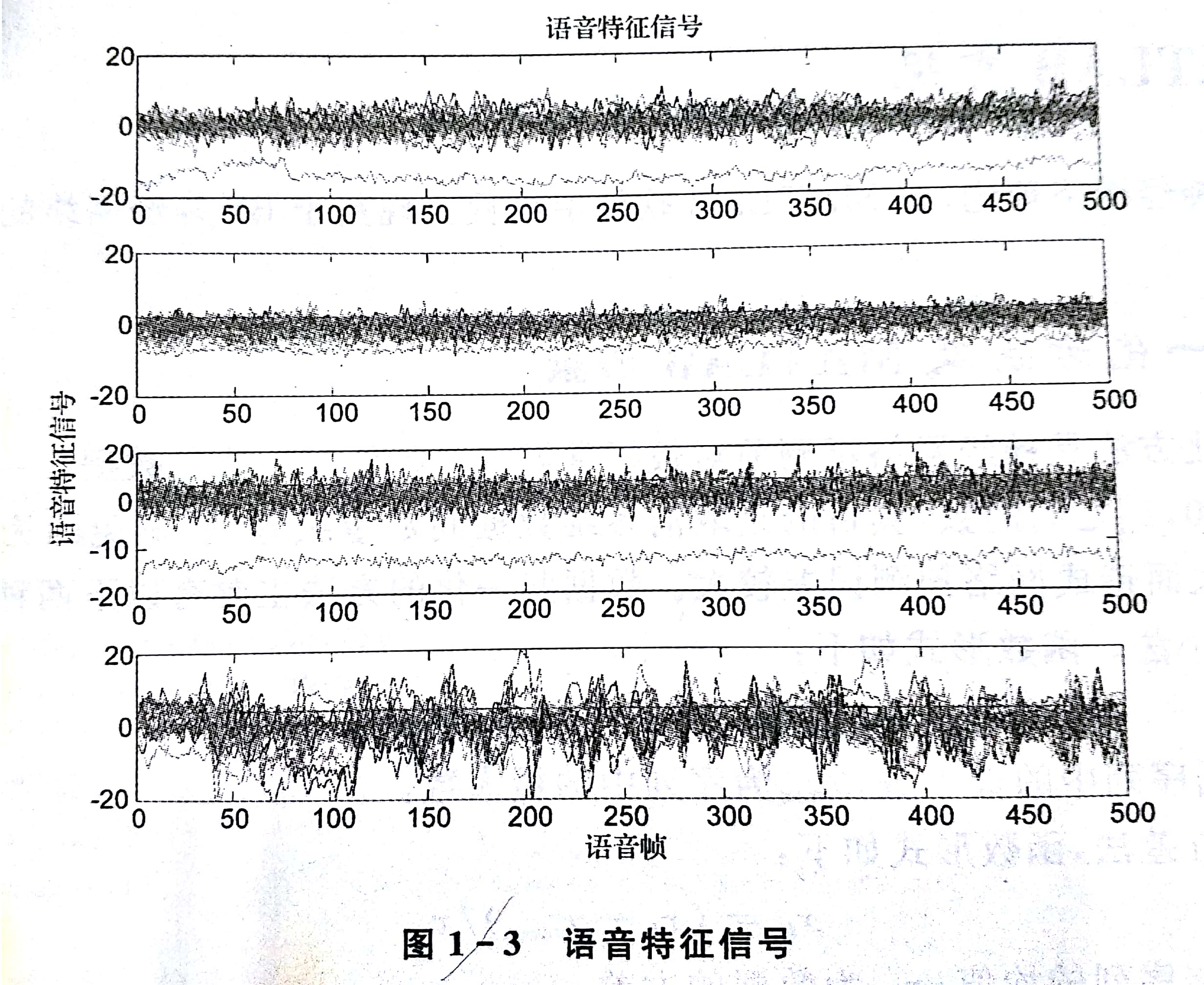三、模型建立
基于BP神经网络的语音特征信号分类算法包括BP神经网络构建、BP神经网络训练和BP神经网络分类三步。算法流程如下：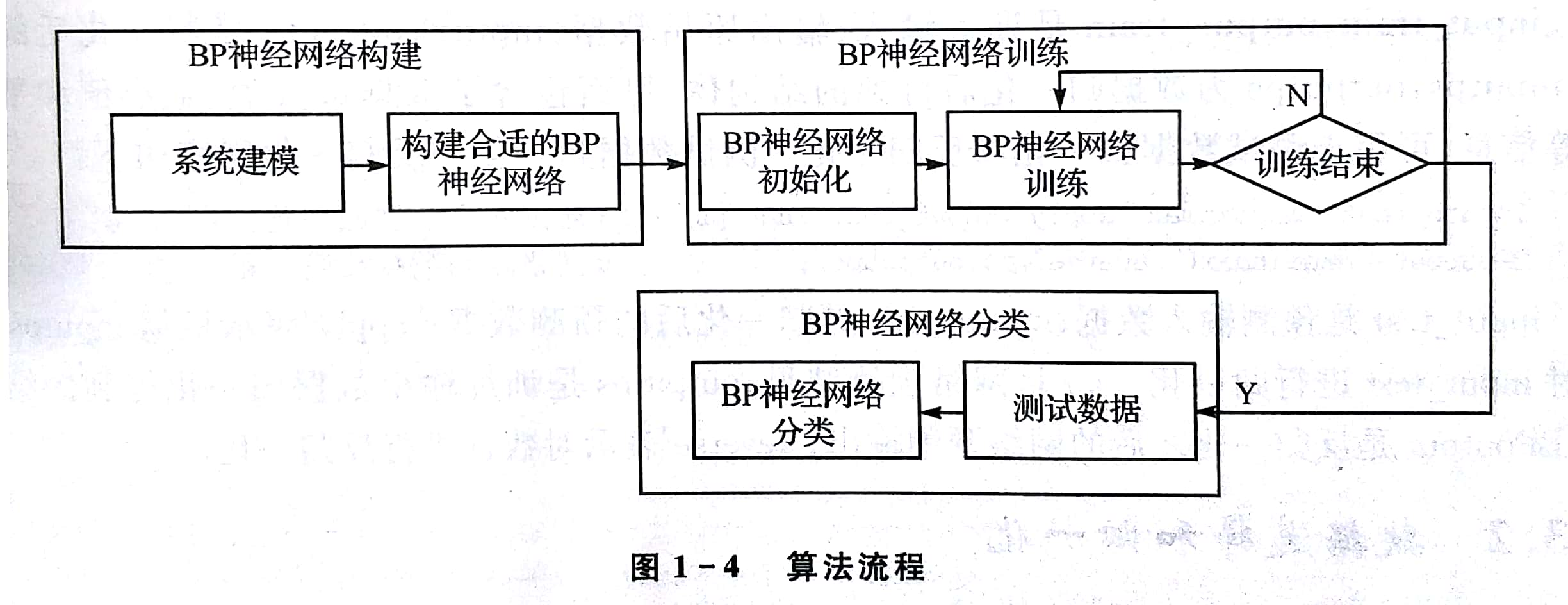BP神经网路构建根据系统输入输出数据特点确定BP神经网络的结构，由于语音特征输入信号有24维，待分类的语音信号共有四类，所以BP神经网络的结构维24-25-4，即输入层油24个节点，隐含层有25个节点，输出层有4个节点。
BP神经网络用训练数据训练BP神经网络。共有2000组语音特征信号，从中随机选择1500组数据作为训练数据训练网络，500组数据作为测试网络测试网络分类能力。
BP神经网络分类用训练好的神经网络对测试数据所属语音类别进行分类。
下面是使用matlab实现基于BP神经网络的语音特征信号分类算法：
基于BP神经网络的语音特征信号分类算法的matlab实现
四、案例扩展
1.隐含层节点数
BP神经网络的隐含层节点数对BP神经网络预测精度有较大影响：
节点数太少，网络不能很好的学习，需要增加训练次数，训练的精度也受影响节点数太多，训练连时间增加，网络容易过拟合。
最佳隐含层节点数选择可参考如下公式：

其中，n为输入层节点数；l为隐含层节点数；m为输出层节点数；a为0~10之间的常数。
在实际问题中，隐含层节点数首先要参考公式，计算出大致的范围。然后用试凑法找出最佳节点数。
一般来说，BP神经网络误差随着隐含层节点的增加是先减少后增加的趋势。
2.附加动量方法
BP神经网络的采用梯度修正法作为权值和阈值的学习算法，从网络预测误差的负梯度方向修正权值和阈值。没有考虑以前经验的积累。学习过程收敛缓慢。可以采用附加动量的方法来解决。公式为：

式中，w(k),w(k-1),w(k-2)分别为k,k-1,k-2时刻的权值；a为动量学习率
matlab程序为：
% xite,alfa为学习率
w1 = w1_1 + xite * dw1' +alfa * (w1_1 - w1_2);
b1 = b1_1 + xite * db1' +alfa * (b1_1 - b1_2);
w2 = w2_1 + xite * dw2' +alfa * (w2_1 - w2_2);
b2 = b2_1 + xite * db2' +alfa * (b2_1 - b2_2);
3.变学习率学习算法
BP神经网络学习率的取值在[0,1]之间，学习率越大，对权值的修改越大，网络学习速度越快。但是过大的学习率将使权值学习产生震荡，过小的学习率使网络收敛过慢，权值难以趋于稳定。变学习率方法是学习率在BP神经网络的进化初期较大，网络收敛迅速，随着学习过程的进行，学习率不断减小，网络趋于稳定。变学习率公式为：

其中，为最大学习率，为最小学习率，为最大迭代次数，t为当前迭代次数
展开全文matlab 神经网络 基础知识 语音信号
• 基于MATLAB，BP神经网络用于二分类，以蚊子分类为例。内有实验代码，实验运行结果。matlab
• 主要介绍了用Python实现BP神经网络（附代码），文中通过示例代码介绍的非常详细，对大家的学习或者工作具有一定的参考学习价值，需要的朋友们下面随着小编来一起学习学习吧
• 包含两个图像分类的资源 1.bp算法 用matlab实现 图像...2.基于神经网络的图像分类，数据资源是基于CIFAR-10，内含训练测试数据（batch），是唐宇迪深度学习入门课程的修改代码，python3版本 源码公开，仅供学习使用。
• 手动搭建了一个bp神经网路，对乳腺癌数据集进行分类。数据集从sklearn中获得，神经网络用numpy手动搭建。手动搭建了一个bp神经网路，对乳腺癌数据集进行分类。数据集从sklearn中获得，神经网络用numpy手动搭建。
• 文件说明： 1、NeuralNetwork_BP_Classification.m - 分类 2、NeuralNetwork_BP_Regression.m - 回归
• ## 用BP神经网络解决简单的分类问题

万次阅读 多人点赞 2018-03-09 20:13:21
有关于BP神经网络原理前人之述备矣，这里就暂且略过。从一年前第一次接触机器学习到现在，已经学习很多类似的智能学习算法，无论是遗传算法、群算法、模拟退火算法还是神经网络算法，在有监督的学习算法中，无非就是...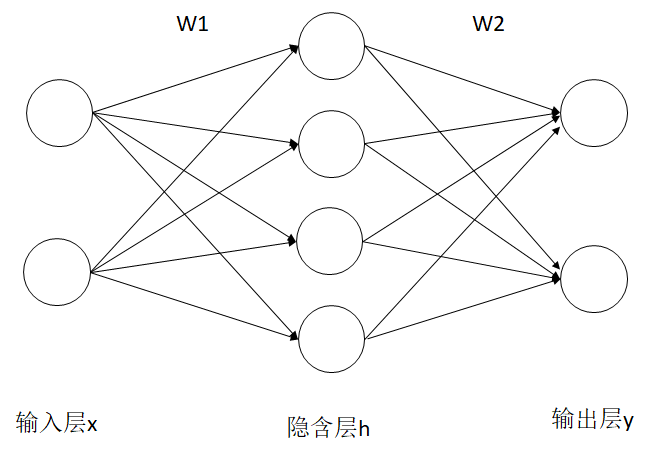有关于BP神经网络原理前人之述备矣，这里就暂且略过。从一年前第一次接触机器学习到现在，已经学习很多类似的智能学习算法，无论是遗传算法、群算法、模拟退火算法还是神经网络算法，在有监督的学习算法中，无非就是设计一个标准和进化方式，让结果与标准之间的误差越来越少，直到误差缩小到允许的范围内并收敛，我们谓之学习成功了。BP神经网络也是这样的，在BP神经网络学习的目标就是输出层输出的结果与真实值的差距尽可能的少。而在更新参数时实际上用的是梯度下降的方法，所以需要计算一些偏导数。而在解决分类问题中，每一层的神经元数目的设定，可以根据数据选择，我目前的建议是输入数据是多少维，输入层就设置多少个神经元，每一个输入层神经元对应一个维度上的分量。数据总共要分成多少类，输出层就设置多少个神经元，比如假设要分为四类，可以设置输入这四类数据时期望的输出分别为[0 0 0 1],[0 0 1 0],[0 1 0 0 ]和[1 0 0 0]。至于隐含层的数目，我目前也不确切的知道应该设置多少个神经元，需要进一步的学习。这两点只是我今天想到的，是一种有点朴素的想法而已。
下面是一个用BP神经网络解决分类问题的MATLAB实现。
clc,clear

traindata = data(:,1:2000);
testdata = data(:,2001:3000);

insize = 2;%输入层神经元数目
hidesize = 10;%隐含层神经元数目
outsize = 2;%输出层神经元数目

yita1 = 0.001;%输入层到隐含层之间的学习率
yita2 = 0.001;%隐含层到输出层之间的学习率

W1 = rand(hidesize,insize);%输入层到隐含层之间的权重
W2 = rand(outsize,hidesize);%隐含层到输出层之间的权重
B1 = rand(hidesize,1);%隐含层神经元的阈值
B2 = rand(outsize,1);%输出层神经元的阈值

Y = zeros(2,2000);%期望输出
for i = 1:2000
y = zeros(2,1);
if traindata(3,i)==1
y = [1;0];
else
y = [0;1];
end
Y(:,i) = y;
end

loop = 1000;
E = zeros(1,loop);
for loopi = 1:loop

for i = 1:2000
x = traindata(1:2,i);

hidein = W1*x+B1;%隐含层输入值
hideout = zeros(hidesize,1);%计算隐含层输出值
for j = 1:hidesize
hideout(j) = sigmod(hidein(j));
end

yin = W2*hideout+B2;%输入层输入值
yout = zeros(outsize,1);%输出层输出值
for j = 1:outsize
yout(j) = sigmod(yin(j));
end

e = yout-Y(:,i);%输出层计算结果误差
E(loopi) = e(1)+e(2);
%%%%%%%%%%%%%%%%%%%%%%%%%%%%%%%%%%%%%%%%%%%%%%
%后向反馈
dB2 = zeros(outsize,1);%误差对输出层阈值求偏导，计算阈值变化量
for j = 1:outsize
dB2 = sigmod(yin(j))*(1-sigmod(yin(j)))*e(j)*yita2;
end

%隐含层与输出层之间的权重的变化量
dW2 = zeros(outsize,hidesize);
for j = 1:outsize
for k = 1:hidesize
dW2(j,k) = sigmod(yin(j))*(1-sigmod(yin(j)))*hideout(k)*e(j)*yita2;
end
end

%隐含层阈值变化量
dB1 = zeros(hidesize,1);
for j = 1:hidesize
tempsum = 0;
for k = 1:outsize
tempsum = tempsum + sigmod(yin(k))*(1-sigmod(yin(k)))*W2(k,j)*sigmod(hidein(j))*(1-sigmod(hidein(j)))*e(k)*yita1;
end
dB1(j) = tempsum;
end

%输入层到隐含层的权重变化量
dW1 = zeros(hidesize,insize);
for j = 1:hidesize
for k = 1:insize
tempsum = 0;
for m = 1:outsize
tempsum = tempsum + sigmod(yin(m))*(1-sigmod(yin(m)))*W2(m,j)*sigmod(hidein(j))*(1-sigmod(hidein(j)))*x(k)*e(m)*yita1;
end

dW1(j,k) = tempsum;

end

end

W1 = W1-dW1;
W2 = W2-dW2;
B1 = B1-dB1;
B2 = B2-dB2;

end

if mod(loopi,100)==0
loopi
end

end

plot(E);

% %查看训练效果
% tempyout = zeros(2,1000);
% for i = 1:1000
%     x = testdata(1:2,i);
%
%     hidein = W1*x+B1;%隐含层输入值
%     hideout = zeros(hidesize,1);%隐含层输出值
%     for j = 1:hidesize
%         hideout(j) = sigmod(hidein(j));
%     end
%
%     yin = W2*hideout+B2;%输出层输入值
%     yout = zeros(outsize,1);
%     for j = 1:outsize
%         yout(j) = sigmod(yin(j));
%     end
%
%     tempyout(:,i) = yout;
%
%     if yout(1)>yout(2)
%         scatter(x(1),x(2),'r')
%         hold on;
%     else
%         scatter(x(1),x(2),'g')
%         hold on;
%     end
%
%
% end
当迭代1000次时的训练效果和分类效果分别如下
训练效果：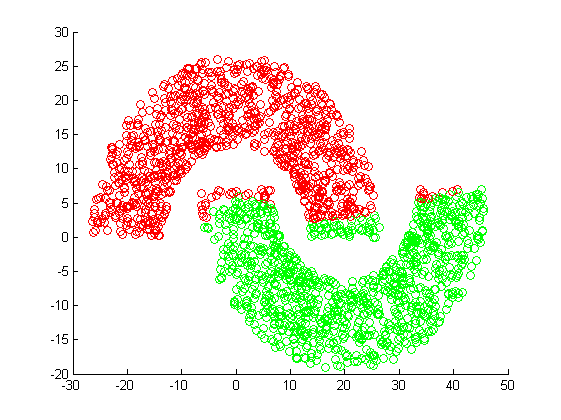测试效果：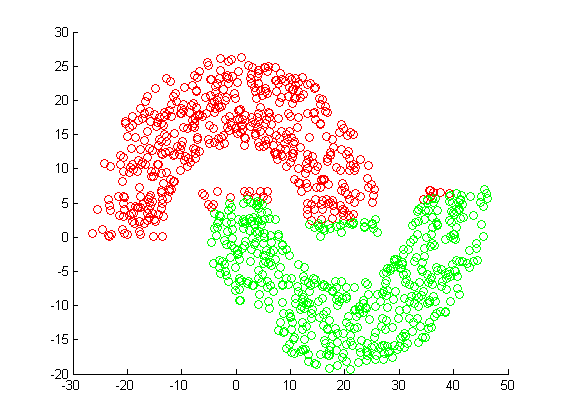我们可以看到当迭代次数为1000次时，分类的正确率已经比较高了，只有少量点分错。
而当迭代次数达到10000次时的训练效果如下图所示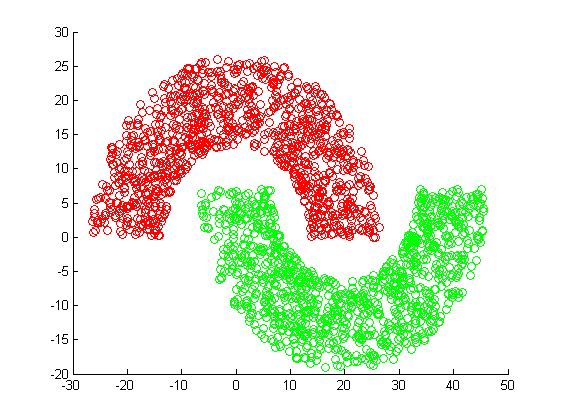测试效果：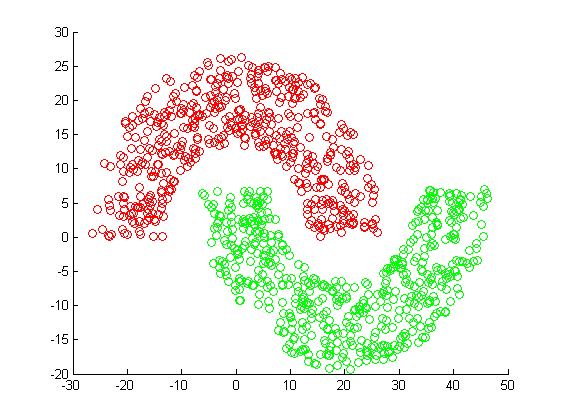而当迭代次数达到10000次时，对于图中的数据正确率已经到达了100%。当然在实际情况中，这种结果并不一定好，有可能会出现过拟合的情况。
本实例中所用到的数据可以从链接：https://pan.baidu.com/s/1Uxt9RurmBraFR-SjGquoww 密码：beb1下载。

----------------------------------------补充--------------------------------------------------
有好多同志私信或留言说MATLAB提示缺少sigmod函数，本以为大家都知道的，看来在这里还是需要补充一下。这是属于BP神经网络的基本原理部分，我开头的一句“前人之述备矣”好像是有点不负责任的嫌疑。这个函数就是那个常用的激活函数：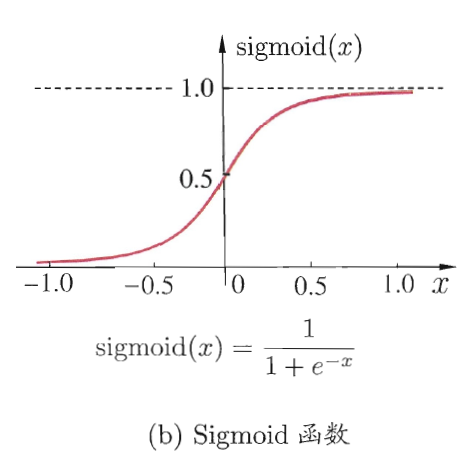写这么一个函数就可以了
function [ y ] = sigmod( x )
% 激活函数Sigmod，用于神经网络
%   鸿武六年三月七日
y = 1/(1+exp(-x));

end
测试数据的话上面的那个百度云链接好像还没有失效。

如有不当之处，欢迎通过QQ进行深入交流，同时也欢迎通过微信打赏的方式对博主进行支持。
展开全文MATLAB 机器学习 人工智能
• BP(back propagation)神经网络是1986年由Rumelhart和...我们用BP神经网络来解决非线性的二分类问题 测试样本： 我们运用Python构建BP神经网络，来解决这个非线性分类问题，代码如下 import numpy as np imporpython 非线性
• 1.BP神经网络简介 BP神经网络是1986年由Rumelhart和McClelland为首的科学家提出的概念，是一种按照逆向传播算法训练的多层前馈神经网络，是目前应用最广泛的神经网络。 优点：具有任意复杂的模式分类能力和优良的...神经网络公式推导 神经网络
• 本文主要内容包括： (1) 介绍神经网络基本原理，(2) AForge.NET实现前向神经网络的方法，(3) Matlab实现前向神经网络的方法 。   第0节、引例   本文以Fisher的Iris数据集作为神经网络程序的测试...matlab
• 本文介绍了利用BP神经网络实现对不同半径的圆进行多分类（3分类），特征即为圆的半径。 输入层12节点，一个6节点的隐藏层，输出层3个节点。
• BP神经网络是一种多层前馈神经网络，网络信号向前传递，误差反向传播。在已知的神经网络基础上，通过北航出版社开源MATLAB神经网络开源训练和代码库来学习在MATLAB上建立和学习BP神经网络。 %% 该代码为基于BP网络...
• 使用tensorflow实现鸢尾花分类，详细易懂。
• ## BP神经网络简单代码分析

千次阅读 多人点赞 2019-10-14 04:26:41
文章目录BP神经网络的构建 BP神经网络的构建 BP网是一种前馈多层（一般都选用3层）网络。理论已经证明一个三层网络可以无限近似任意连续函数。 现在，我们来看一个构建BP神经网络的matlab代码： % BP网络 % ...
• BP神经网络结构初始化   根据语音特征信号特点确定BP神经网络的结构为24-25-4，随机初始化BP神经网络权值和阈值。 %% 网络结构初始化 innum=24; midnum=25; outnum=4; %权值初始化 w1=rands(midnum...MATLAB NetWork
• 在人工神经网络的实际应用中，BP网络广泛应用于函数逼近、模式识别/分类、数据压缩等，80%~90%的人工神经网络模型是采用BP网络或它的变化形式，它也是前馈网络的核心部分，体现了人工神经网络最精华的部分。MATLAB
• 本篇文章主要介绍了PyTorch上搭建简单神经网络实现回归和分类的示例，小编觉得挺不错的，现在分享给大家，也给大家做个参考。一起跟随小编过来看看吧...